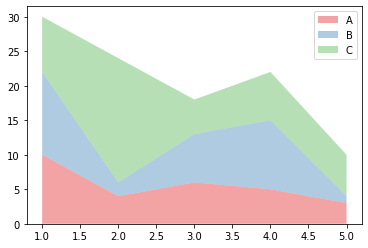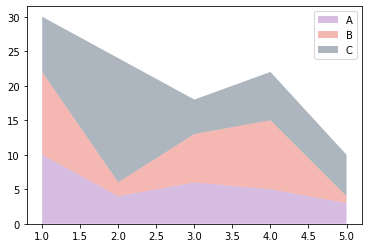You can change the colors in your stacked area chart with the `colors` parameter of the `stackplot()` function. Here I propose 2 solutions: the first one is to use a common color palette, the second is to pick up your colors one by one.

``````# libraries
import numpy as np
import matplotlib.pyplot as plt
import seaborn as sns

# Your x and y axis
x = range(1,6)
y = [ [10,4,6,5,3], [12,2,7,10,1], [8,18,5,7,6] ]

# use a known color palette
pal = sns.color_palette("Set1")
plt.stackplot(x,y, labels=['A','B','C'], colors=pal, alpha=0.4 )
plt.legend(loc='upper right')
plt.show()